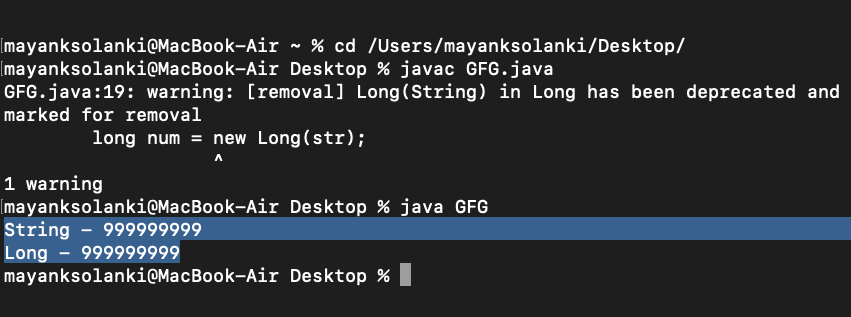# Java Program to Convert String to Long

• Difficulty Level : Expert
• Last Updated : 03 May, 2022

There are many methods for converting a String to a Long data type in Java which are as follows:

1. Using the parseLong() method of the Long class
2. Using valueOf() method of long class
3. Using constructor of Long class

Illustrations:

```Input  : String = "20"
Output : 20```
```Input  : String = "999999999999"
Output : 999999999999```

### Method 1: Using Long.parseLong() method of Long Class

Long.parseLong() method is a method in which all the characters in the String must be digits except the first character, which can be a digit or a minus ‘-‘.

Syntax:

`Long varLong=Long.parseLong(str);`

Example:

## Java

 `// Java Program to convert String to Long``// using parseLong() Method` `// Class``public` `class` `GFG {` `    ``// Main driver method``    ``public` `static` `void` `main(String args[])``    ``{``        ``// Creating a custom string``        ``String str = ``"999999999999"``;``        ``System.out.println(``"String - "` `+ str);` `        ``// Converting into Long``        ``long` `varLong = Long.parseLong(str);` `        ``// Printing String as Long``        ``System.out.println(``"Long - "` `+ varLong);``    ``}``}`

Output

```String - 999999999999
Long - 999999999999```

### Method 2: Using valueOf() method of Long Class

valueOf() method of the Long class is a method that converts the String to a long value. Similar to parseLong(String) method, this method also allows minus ‘-‘ as a first character in the String.

Syntax:

`long varLong = Long.valueOf(str);`

Example:

## Java

 `// Java Program to Convert String to Long``// Using valueOf() Method` `// Class``public` `class` `GFG {` `    ``// Main driver method``    ``public` `static` `void` `main(String args[])``    ``{``        ``// Creating custom string``        ``String str = ``"999999999999"``;` `        ``// Printing the above string``        ``System.out.println(``"String - "` `+ str);` `        ``// Converting into Long data type``        ``long` `varLong = Long.valueOf(str);` `        ``// Printing String as Long``        ``System.out.println(``"Long - "` `+ varLong);``    ``}``}`

Output

```String - 999999999999
Long - 999999999999```

### Method 3: Using Constructor of Long class

The long class has a constructor which allows the String argument and creates a new Long object representing the specified string in the equivalent long value.

Example:

## Java

 `// Java Program to Convert String to Long``// Using Constructor of Long class` `// Importing required classes``import` `java.io.*;``import` `java.util.*;` `// Class``class` `GFG {` `    ``// Main driver method``    ``public` `static` `void` `main(String[] args)``    ``{``        ``// Custom input string``        ``String str = ``"999999999"``;``        ``System.out.println(``"String - "` `+ str);` `        ``// Converting above string to long``        ``// using Long(String s) constructor``        ``long` `num = ``new` `Long(str);` `        ``// Printing the above long value``        ``System.out.println(``"Long - "` `+ num);``    ``}``}`

Output:My Personal Notes arrow_drop_up